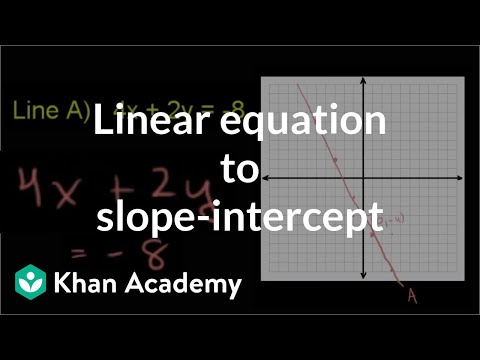Video

# Worked examples: slope-intercept intro (Full video)

Description: Worked examples practicing the relationship between slope, y-intercept, and linear equation in slope-intercept form. Let's do some practice examples from our intro to slope y intercept exercise. What is the slope of y is equal to negative four x minus three? So you might already recognize, this is in slope intercept form.

### Other videos you might be interested in### Ratio and Proportion in Grades 6-8: Connections to College and Career Skills (Full video)

#### The Hunt Institute